TRIGONOMETRIC RATIOS OF ANGLES OF ANY MAGNITUDE - PART 2Learning Objectives - This is what you must know after studying the lecture and doing the practice problems!

1.   Find reference angles in degrees and radians of given angles.
2.   Find the exact values of trigonometric ratios of integer multiples of "special" angles without a calculator.We are going to continue our discussion of trigonometric ratios of angles of any magnitude. Besides the quadrantal angles and their integer multiples, there is is one other set of angles that appear often in mathematics, physics, and engineering and those are the integer multiples of the "special" angles 30o, 45o, and 60o.

It is common practice to teach trigonometry students how to find the EXACT values of the trigonometric ratios of integer multiples of special angles without using the calculator.

The following integer multiples occur frequently. You must memorize them. You must also memorize their radian equivalents.

Often used integer multiples of 30o

5(30o) = 150o and 7(30o) = 210o and 11(30o) = 330o

NOTE:Often used integer multiples of 45o

3(45o) = 135o and 5(45o) = 225o and 7(45o) = 315o

NOTE: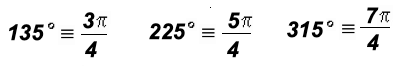Often used integer multiples of 60o

2(60o) = 120o and 4(60o) = 240o and 5(60o) = 300o

Please note that 120o, 240o, and 300o are also integer multiples of 30o. However, any time 60o divides evenly into an angle measure, it is considered an integer multiple of 60o.

NOTE:Reference Angles - see #1, 2, and 3 in the "Examples" document

Before we find the EXACT values of their trigonometric ratios without using the calculator, a discussion of reference angles is necessary.

Reference angles are positive acute (less than 90o) angles that lie between the terminal side of some angle and the horizontal axis in a coordinate system.

How to find a Reference Anglefor some Angle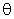:

Assume thatis positive and between 0o and 360o (or 0 and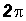).

1. Ifis a first-quadrant angle, then the reference angleequals.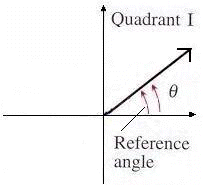2. Ifis a second-quadrant angle, then the reference angleequals 180o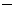or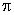.3. Ifis a third-quadrant angle, then the reference angleequals180o or.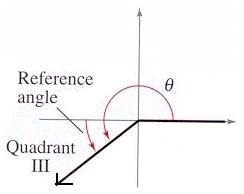4. Ifis a fourth-quadrant angle, then the reference angleequals 360oor.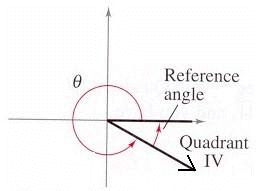Four Characteristics of Reference Angles:

The follwoing characteristics of reference angles will be stated without a formal proof. They should be evident from the discussion found under the link "Point of Interest 2" in the Learning Materials #4 in the MyOpenMath course.

1. The trigonometric ratio of an angleand that of its reference anglehave the same ABSOLUTE value.

2. Negative angles have the same reference angles as their positive counterparts.

3. Coterminal angles have the same reference angle.

4. Quadrantal angles do not have reference angles.

Strategy for Finding EXACT Values of Trigonometric Ratios of Integer Multiples of Special Angles WITHOUT a Calculator - see #4 through 14 in the "Examples" document

Assume that angleis an integer multiple of a "special" angle.

Step 1:

Find the reference angleof the given angle. Might have to use one or more of the four characteristics of reference angles.

Step 2:

Find the value of the given trigonometric ratio of the reference anglefrom Step 1.

Step 3:

Find the value of the trigonometric ratio of the given anglewith the help of the following:

• the value found in Step 2
• one or more of the four characteristics of reference angles
• "All Students Take Calculus" on the given trigonometric ratio of angleNOTE: Some students who have had trigonometry in the past might have memorized the following unit circle.It is NOT a good idea to memorize the coordinates of the points in the picture above! Using Reference Angles is necessary for later concepts as well and anyone who refuses to learn it now will be in trouble later on! So please, do not use your unit circle coordinates.